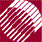ECE532 Biomedical Optics © 1998 Steven L. Jacques, Scott A. Prahl Oregon Graduate Institute

# Experiment: Calibrating a light bulb

We shall calibrate the radiant power, P [W], of a light bulb by immersing it within a cup of black water and capturing all the radiant energy, Q [J], generated during an exposure time, t [s]. Measuring the temperature rise of the water will yield the power of the light bulb.

• Prepare a cup of water by adding India ink so it is very black. (V = 100 ml final volume)

• Measure the initial temperature of the water. (Ti = 22.50 °C)

• Immerse a small 10 W light bulb within the cup of water and turn it on. No light escapes the black cup of water. All energy is absorbed and heats the water.

• Turn the light off after one minute. (t = 60 s)

• Stir the water to uniformly distribute the heat and measure the final temperature rise using a digital thermometer. (Tf = 25.287 °C)

• For water:
• density = rho = 1 [g/cm3]
• heat capacity = C = 4.18 J/(g °C)
• rho*C = 4.18 J/(cm3 °C)
• We shall neglect the heat capacity and mass of the cup itself in this calculation. The cup causes a change in the apparent value of the product rho*C*V. See Discussion of cup for guide to considering this additional factor in a real experiment.

• Total energy::
Q = (Tf - Ti)*rho*C*V = (25.287 - 25.00)*1*4.18*500 = 600 J

• Lightbulb power,
P = Q/t = 600/60 = 10 W

The radiant power P of the light bulb is 10 W.